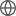Old Web
English
# The ALMA Spectroscopic Survey in the HUDF: A Search for [CII] Emitters at $6 \leq z \leq 8$.
The ALMA Spectroscopic Survey in the Hubble Ultra Deep Field (ASPECS) Band 6 scan (212-272 GHz) covers potential [CII] emission in galaxies at $6\leq z \leq8$ throughout a 2.9 arcmin$^2$ area. By selecting on known Lyman-$\alpha$ emitters (LAEs) and photometric dropout galaxies in the field, we perform targeted searches down to a 5$\sigma$ [CII] luminosity depth $L_{\mathrm{[CII]}}\sim2.0\times10^8$ L$_{\odot}$, corresponding roughly to star formation rates (SFRs) of $10$-$20$ M$_{\odot}$ yr$^{-1}$ when applying a locally calibrated conversion for star-forming galaxies, yielding zero detections. While the majority of galaxies in this sample are characterized by lower SFRs, the resulting upper limits on [CII] luminosity in these sources are consistent with the current literature sample of targeted ALMA observations of $z=6$-$7$ LAEs and Lyman-break galaxies (LBGs), as well as the locally calibrated relations between $L_{\mathrm{[CII]}}$ and SFR -- with the exception of a single [CII]-deficient, UV luminous LBG. We also perform a blind search for [CII]-bright galaxies that may have been missed by optical selections, resulting in an upper limit on the cumulative number density of [CII] sources with $L_{\mathrm{[CII]}}>2.0\times10^8$ L$_{\odot}$ ($5\sigma$) to be less than $1.8\times10^{-4}$ Mpc$^{-3}$ (90% confidence level). At this luminosity depth and volume coverage, we present an observed evolution of the [CII] luminosity function from $z=6$-$8$ to $z\sim0$ by comparing the ASPECS measurement to literature results at lower redshift.